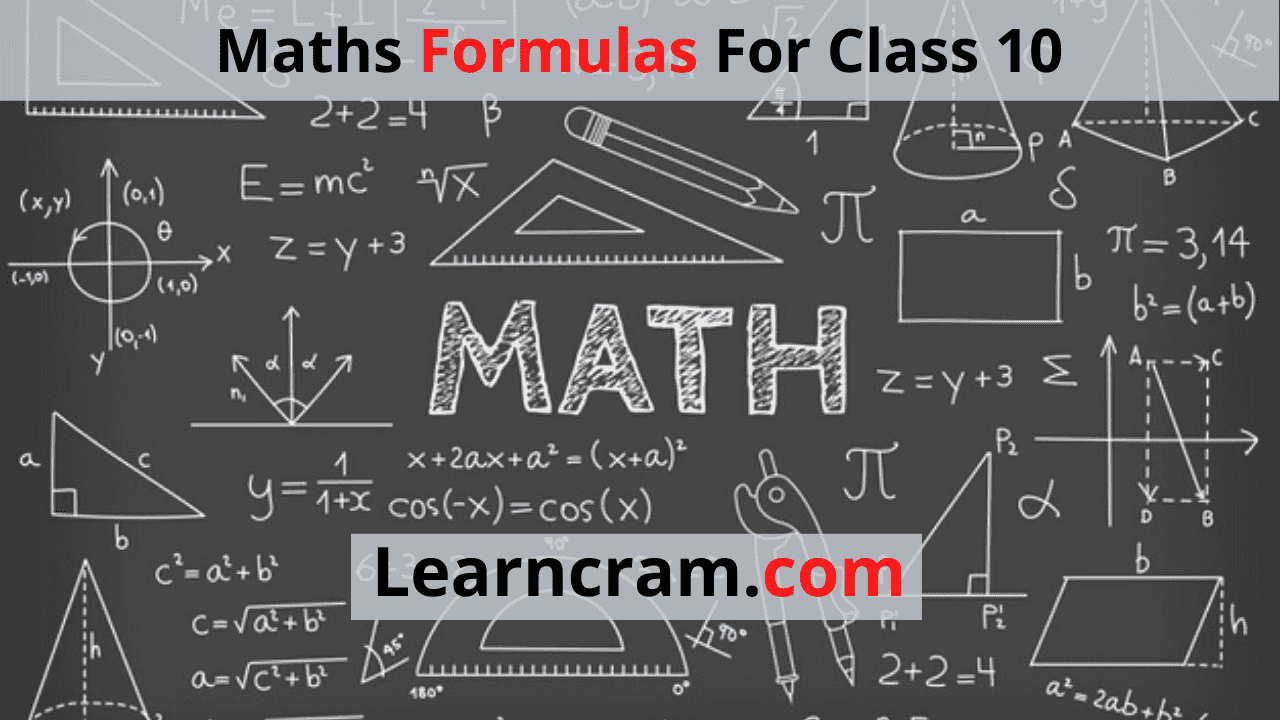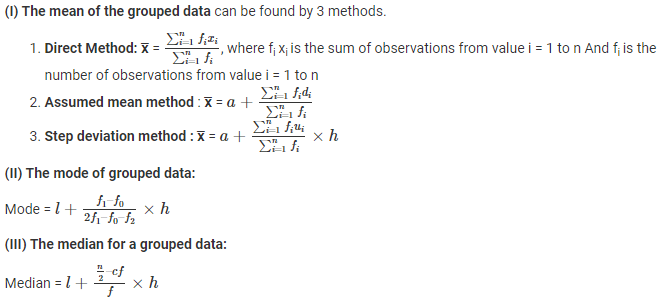# Maths Formulas For Class 10

For some Maths can be fun and for some, it can be a nightmare. Maths Formulas are difficult to memorise and we have curated a list of Maths Formulas for Class 10 just for you. You can use this as a go-to sheet whenever you want to prepare Class 10 Maths Formulas. Students can get Formulas on Algebra, Calculus, and Geometry that can be useful in your preparation and help you do your homework.

Here in this article, we have listed basic Maths formulas so that you can learn the fundamentals of Maths. Our unique way of solving Maths Problems will make you learn how the equation came into existence instead of memorizing it. Solve all the important problems and questions in Maths with the Best Maths Formulas for Class 10.

Feel free to directly use the best Maths formulas during your homework or exam preparation. You need to know the list of Class 10 formulas as they will not just be useful in your academic books but also in your day to day lives.

Remember the Maths Formulas in a smart way by making use of our list. You can practice Questions and Answers based on these Class 10 Maths Formulas. Students can get basic Maths formulas Free PDF Download for Class 10. Candidates can use the handy learning aid Maths Formulas PDF to have in depth knowledge on the subject as per the Latest CBSE Syllabus.

CBSE Class 10 Maths Formulas according to the Chapters are prepared by subject experts and you can rely on them during your preparation. Click on the topic you wish to prepare from the list of formulas prevailing.

### Linear Equations

 One Variable ax+b=0 a≠0 and a&b are real numbers Two variable ax+by+c = 0 a≠0 & b≠0 and a,b & c are real numbers Three Variable ax+by+cz+d=0 a≠0 , b≠0, c≠0 and a,b,c,d are real numbers

### Pair of Linear Equations in two variables:

a1x+b1+c1=0
a2x+b2+c2=0

Where

• a1, b1, c1, a2, b2, and c2 are all real numbers and
• a12+b12 ≠ 0 & a2+ b22 ≠ 0

It should be noted that linear equations in two variables can also be represented in graphical form.

### Algebra or Algebraic Equations

The standard form of Quadratic Equations

ax2+bx+c=0 where a ≠ 0
And x = [-b ± √(b2 – 4ac)]/2a

### Algebraic formulas:

• (a+b)= a+ b+ 2ab
• (a-b)= a+ b– 2ab
• (a+b) (a-b) = a– b2
• (x + a)(x + b) = x2 + (a + b)x + ab
• (x + a)(x – b) = x2 + (a – b)x – ab
• (x – a)(x + b) = x2 + (b – a)x – ab
• (x – a)(x – b) = x2 – (a + b)x + ab
• (a + b)3 = a3 + b3 + 3ab(a + b)
• (a – b)3 = a3 – b3 – 3ab(a – b)
• (x + y + z)2 = x2 + y2 + z2 + 2xy + 2yz + 2xz
• (x + y – z)2 = x2 + y2 + z2 + 2xy – 2yz – 2xz
• (x – y + z)2 = x2 + y2 + z2 – 2xy – 2yz + 2xz
• (x – y – z)2 = x2 + y2 + z2 – 2xy + 2yz – 2xz
• x3 + y3 + z3 – 3xyz = (x + y + z)(x2 + y2 + z2 – xy – yz -xz)
• x+ y2 =½ [(x + y)2 + (x – y)2]
• (x + a) (x + b) (x + c) = x3 + (a + b +c)x2 + (ab + bc + ca)x + abc
• x3 + y3= (x + y) (x2 – xy + y2)
• x3 – y3 = (x – y) (x2 + xy + y2)
• x2 + y2 + z2 -xy – yz – zx = ½ [(x-y)2 + (y-z)2 + (z-x)2]

### Basic formulas for powers

• px p= pm+n
• {pm}⁄{pn} = pm-n
• (pm)= pmn
• p-m = 1/pm
• p1 = p
• P= 1

### Arithmetic Progression(AP) Formulas

If a1, a2, a3, a4, a5, a6, are the terms of AP and d is the common difference between each term, then we can write the sequence as; a, a+d, a+2d, a+3d,

### Algebraic formulas:

• (a+b)= a+ b+ 2ab
• (a-b)= a+ b– 2ab
• (a+b) (a-b) = a– b2
• (x + a)(x + b) = x2 + (a + b)x + ab
• (x + a)(x – b) = x2 + (a – b)x – ab
• (x – a)(x + b) = x2 + (b – a)x – ab
• (x – a)(x – b) = x2 – (a + b)x + ab
• (a + b)3 = a3 + b3 + 3ab(a + b)
• (a – b)3 = a3 – b3 – 3ab(a – b)
• (x + y + z)2 = x2 + y2 + z2 + 2xy + 2yz + 2xz
• (x + y – z)2 = x2 + y2 + z2 + 2xy – 2yz – 2xz
• (x – y + z)2 = x2 + y2 + z2 – 2xy – 2yz + 2xz
• (x – y – z)2 = x2 + y2 + z2 – 2xy + 2yz – 2xz
• x3 + y3 + z3 – 3xyz = (x + y + z)(x2 + y2 + z2 – xy – yz -xz)
• x+ y2 =½ [(x + y)2 + (x – y)2]
• (x + a) (x + b) (x + c) = x3 + (a + b +c)x2 + (ab + bc + ca)x + abc
• x3 + y3= (x + y) (x2 – xy + y2)
• x3 – y3 = (x – y) (x2 + xy + y2)
• x2 + y2 + z2 -xy – yz – zx = ½ [(x-y)2 + (y-z)2 + (z-x)2]

### Basic formulas for powers

• px p= pm+n
• {pm}⁄{pn} = pm-n
• (pm)= pmn
• p-m = 1/pm
• p1 = p
• P= 1

### Arithmetic Progression(AP) Formulas

If a1, a2, a3, a4, a5, a6, are the terms of AP and d is the common difference between each term, then we can write the sequence as; aa+d, a+2d, a+3d, a+4d, a+5d,….,nth term… where a is the first term. Now, nth term for arithmetic progression is given as;

nth term = a + (n-1) d

Sum of nth term in Arithmetic Progression;

Sn = n/2 [a + (n-1) d]

### Trigonometry Formulas For Class 10

Trigonometry maths formulas for Class 10 covers three major functions Sine, Cosine and Tangent for a right-angle triangle. Also, in trigonometry, the functions sec, cosec and cot formulas can be derived with the help of sin, cos and tan formulas.

Let a right-angled triangle ABC is right-angled at point B and have ∠θTrigonometry Table:Other Trigonometric formulas:

• sin(90° – θ) = cos θ
• cos(90° – θ) = sin θ
• tan(90° – θ) = cot θ
• cot(90° – θ) = tan θ
• sec(90° – θ) = cosecθ
• cosec(90° – θ) = secθ
• sin2θ + cos2 θ = 1
• secθ = 1 + tan2θ for 0° ≤ θ < 90°
• Cosecθ = 1 + cot2 θ for 0° ≤ θ ≤ 90°

### Circles Formulas For Class 10

• Circumference of the circle = 2 π r
• Area of the circle = π r2
• Area of the sector of angle θ = (θ/360) × π r2
• Length of an arc of a sector of angle θ = (θ/360) × 2 π r

(r = radius of the circle)

### Surface Area and Volumes Formulas For Class 10

The common formulas from the surface area and volumes chapter in 10th class include the following:

• Sphere Formulas
 Diameter of sphere 2r Circumference of Sphere 2 π r Surface area of sphere 4 π r2 Volume of Cylinder 4/3 π r2
• Cylinder Formulas
 Circumference of Cylinder 2 πrh Curved surface area of Cylinder 2 πr2 Total surface area of Cylinder Circumference of Cylinder + Curved surface area of Cylinder = 2 πrh + 2 πr2 Volume of Cylinder π r2 h
• Cone Formulas
 Slant height of cone l = √(r2 + h2) Curved surface area of cone πrl Total surface area of cone πr (l + r) Volume of cone ⅓ π r2 h
• Cuboid Formulas
 Perimeter of cuboid 4(l + b +h) Length of the longest diagonal of a cuboid √(l2 + b2 + h2) Total surface area of cuboid 2(l×b + b×h + l×h) Volume of Cuboid l × b × h

Here, l = length, b = breadth and h = height In case of Cube, put l = b = h = a, as cube all its sides of equal length, to find the surface area and volumes.

### Statistics Formulas for Class 10

In class 10, the chapter statistics mostly deals with finding the mean median and standard deviation of grouped data.

(I) The mean of the grouped data can be found by 3 methods.### FAQs on Class 10 Maths Formulas

1. Where can I get Maths Formulas for Class 10?

You can find the list of all Maths Formulas pertaining to Class 10 from our page. In fact, all the formulas are arranged topic wise as per chapters and you can use them to score better grades in the exam.

2. How do I Learn Class 10 Maths Formulas?

Don’t try to mug up the formulas instead try finding the logic behind it so that it will be easy for you. However, there are some formulas that are hard to derive and you can memorize them. Practice as much as you can to understand the Maths Class 10 Formulas.

3. Is there a Website that provides all Maths formulas for Class 10?

Students can make use of our website to access all the Class 10 Maths Formulas as per the topics to make your learning process effective.

Final Words

We believe that the comprehensive list of basic Maths formulas for Class 10 will make your learning effective. You can simply click on the Topics to view the Class 10 Maths formulas and aid your preparation. If you feel any formula is missing that can be added to our list do drop us a comment and we will add it to the list.